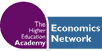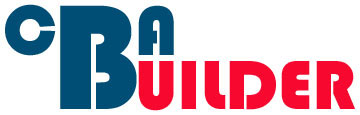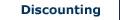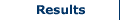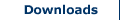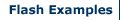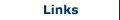Obtaining and Presenting Results

When performing a CBA there are two methods of obtaining and presenting overall results. These are Net Present Value (NPV) and the Benefit Cost Ratio (BCR). Further options do exist, for example, performing a sensitivity analysis. However, NPV and BCR are the most commonly used methods for presenting the results of a CBA.

Net Present Value

The Net Present Value (NPV) of a project is equal to the difference between the present value of the benefits and the present value of the costs. This can be expressed using the following equation: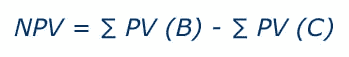Where,
NPV = net present value
PV (B) = present value of the benefits
PV (C) = present value of the costs

This equation is also sometimes written as: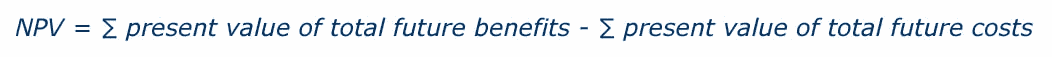The outcome of the CBA when calculated using NPV provides an easy to interpret result for the researcher. If NPV > 0, then it follows that the project has economic justification to go ahead. There is economic justification for the project as long as the sum of the present value of the benefits is greater than the sum of the present value of the costs. The decision rule for NPV can be shown graphically as below.The graph shows that as the alternatives considered generate greater positive differences between benefits and costs the allocation of resources becomes more efficient, offering a socially more optimal outcome. When the difference between benefits and costs is greatest at point Q, this represents the most socially optimal, and most resource efficient outcome.

As alternatives move beyond this point, however, while output continues to increase, the positive difference between benefits and costs reduces and the allocation of resources becomes relatively less efficient than at point Q. However, as benefits are still positive beyond point Q, there may be some occasions where the increased output, albeit with diminishing returns, is chosen as the alternative still provides a positive NPV, but also greater output.

In addition, in reality it may not be possible for the researcher to feasibly identify point Q. In this case the alternative with the greatest gap between benefits and costs will be chosen although it may not necessarily offer the most resource efficient option available should the researcher have the resources to investigate more and more alternatives.

Search CBA Builder:

•